## Friday, September 30, 2011

### 976

976 = 24 x 61.

976 has a square formed by inserting a block of digits inside the original number: 9762 = 952,576.

976 has a representation as a sum of two squares: 976 = 202 + 242.

976 is a divisor of 114 - 1.976-EVIL is a 1989 horror film directed by Robert Englund.

## Thursday, September 29, 2011

### 680

680 = 23 x 5 x 17.

680 is the 15th tetrahedral number. It is the smallest tetrahedral number that is also the sum of two tetrahedral numbers: 680 = 560 + 120.

680 has two representations as a sum of two squares: 680 = 22 + 262 = 142 + 222.

680 is 404 in base 13.

680 can be written as the sum of distinct odd powers of two: 680 = 23 + 25 + 27 + 29.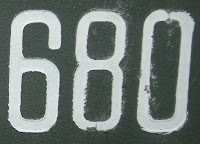WCBM AM 680 is a talk radio station in Baltimore.

## Wednesday, September 28, 2011

### 458

458 = 2 x 229.

458 has a representation as a sum of two squares: 458 = 132 + 172.

458 is a number that does not have any digits in common with its cube: 4583 = 96,071,912.

4582 contains exactly six different digits: 4582 = 209,764.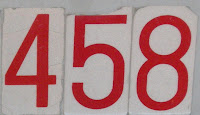The Ferrari 458 Italia is a sports car that was introduced in 2009.

## Tuesday, September 27, 2011

### 156

156 = 22 x 3 x 13. It is a pronic number because it is the product of two consecutive numbers: 156 = 12 x 13.

156 is 1111 in base 5.

156 is the number of graphs with six vertices.A clock will strike 156 times in the course of a day.

## Monday, September 26, 2011

### 452

452 = 22 x 113.

452 has a representation as a sum of two squares: 452 = 142 + 162.

452 is a divisor of 154 - 1.

452 is 381 in base 11 and 318 in base 12. It is 202 in base 15.

452 is the sum of seven nonzero 4th powers.The Petronas Towers in Kuala Lumpur, Malaysia, are 452 meters tall (including antenna spires).

## Friday, September 23, 2011

### 857

857 is a prime number.

857 has a representation as a sum of two squares: 857 = 42 + 292.

857 and 859 form a twin prime pair.

857 is the hypotenuse of a primitive Pythagorean triple: 8572 = 2322 + 8252.

142,857 x 5 = 714,285 = 8572 - 1422, where 857,142 = 142,857 x 6.

857 is 1011202 in base 3. It is 272 in base 19.

857 is the sum of three consecutive primes: 857 = 281 + 283 + 293.Glasenappia 857 is an asteroid discovered in 1916.

Source: Wikipedia

## Thursday, September 22, 2011

### 339

339 = 3 x 113.

339 is the number of ways to divide 5 black and 5 white beads into piles.

3393 = 38,958,219 = (389 - 58 - 2 + 1 + 9)3.

339 is 2324 in base 5 and 1323 in base 6. It is 523 in base 8.

339 is the sum of the squares of three consecutive primes: 339 = 72 + 112 + 132.The Gibson ES-339 is a custom hand-crafted electric guitar.

## Wednesday, September 21, 2011

### 143

143 = 11 x 13.

143 is the smallest brilliant number whose reversal, 341, is a different brilliant number.

143 is an aspiring number.

The last 143 digits of 143143 form a prime number.

143 is the number of three-digit primes.

Every positive integer is the sum of at most 143 seventh powers.

1432 is a divisor of 143143.

143 is the sum of three consecutive primes and the sum of seven consecutive primes: 143 = 43 + 47 + 53 = 11 + 13 + 17 + 19 + 23 + 29 + 31.Vickers Type 143 was a British single-seat biplane designed and built in 1929.

## Tuesday, September 20, 2011

### 247

247 = 13 x 19. Its digits sum to the smallest prime factor: 2 + 4 + 7 = 13.

247 is the smallest possible difference between two integers that together contain each digit exactly once: 247 = 50,123 - 49876.

2472 is one of six square numbers that use the same digits: 2472 = 61,009; 1302 = 16.900; 1402 = 19,600; 3102 = 96,100; 1032 = 10,609; and 3012 = 90,601.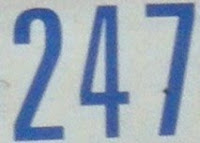247 Eukrate is a large, dark asteroid discovered in 1885.

## Monday, September 19, 2011

### 551

551 = 19 x 29.

551 is the sum of three consecutive primes: 551 = 179 + 181 + 191.

551 is a centered decagonal number.

551 is the number of trees with 12 vertices.

551 is 202102 in base 3 and 20213 in base 4.

551 is a divisor of 303 - 1.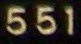551 Ortrud is an asteroid discovered in 1904.

## Friday, September 16, 2011

### 6200

6200 = 23 x 52 x 31.

6200 is 22111122 in base 3 and 8448 in base 9. It is 14070 in base 8.

6200 is a multiple of 8 with a digit sum of 8.

6200 is a divisor of 996 - 1.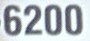The GeForce 6200 from Nvidia is a graphics processing unit introduced in 2004.

## Thursday, September 15, 2011

### 621

621 = 33 x 23.

621 is the number of ways to 9-color a tetrahedron.

621 is 4441 in base 5. It is 515 in base 11.

6212 = 385,641 contains exactly six different digits.

621 is the sum of two distinct positive pentatope numbers: 621 = 126 + 495.The USS Haddock (SSN-621) was a nuclear-powered submarine launched in 1966 and decommissioned in 1993.

## Wednesday, September 14, 2011

### 410

410 = 2 x 5 x 41.

410 has two representations as a sum of two squares: 410 = 72 + 192 = 112 + 172. 410 is the smallest number that can be represented as the sum of the squares of distinct primes in two different ways.

410 is 120012 in base 3, 12122 in base 4, and 1124 in base 7. It is 505 in base 9 and 343 in base 11.

410 is the sum of six consecutive primes: 410 = 57 + 61 + 67 + 71 + 73 + 79.410 is the telephone area code for the Baltimore area of the state of Maryland.

## Tuesday, September 13, 2011

### 1099

1099 = 7 x 157.

1099 is 777 in base 12.

1099 = 1 + 0 + 999 + 99.

1099 and 10992 = 1,207,801 have the same digit sum: 1 + 0 + 9 + 9 = 1 + 2 + 0 + 7 + 8 + 0 + 1 = 19.

1099 is the sum of seven consecutive primes: 1099 = 139 + 149 + 151 + 157 + 163 + 167 + 173.In the United States the 1099 IRS tax form shows how much an independent contractor made from a certain business.

## Monday, September 12, 2011

### 222

222 = 2 x 3 x 37.

222 is the number of integer partitions of 28 into distinct parts.

222 is the smallest number with three prime digits.

The number 2222 + 22222 + 222222 + 1 is a prime.

222 is 1010 in base 6. It is 141 in base 13.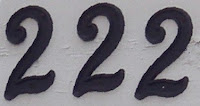Room 222 was a TV show that aired from 1969 to 1974.

## Friday, September 9, 2011

### 8184

8184 = 23 x 3 x 11 x 31.

8184 is a divisor of 975 - 1.

8184 has the representation 213 - 8.

8184 is 1333320 in base 4 and 12203 in base 9. The set of base 4 digits of 8184 equals the set of base 9 digits: {0, 1, 2, 3}. 8184 contains exactly the same digits (with the correct multiplicity) in three different smaller bases.

The product of the digits of 8184 is 8 x 1 x 8 x 4 = 256 = 44, where 4 is the number of digits.

81842 = 66,977,856 contains no digit less than 5.Lego set 8184 is an RC Racer.

## Thursday, September 8, 2011

### 807

807 = 3 x 269.

807!/708! - 1 is a prime number.

807 and 8072 = 651,249 combined use different digits.

807 is the sum of two pentagonal numbers in exactly two ways: 807 = 715 + 92 = 477 + 330.

807 is the sum of 13 positive fifth powers.Telephone area code 807 covers the northwestern portion of the province of Ontario.

## Wednesday, September 7, 2011

### 405

405 = 34 x 5.

405 has a representation as a sum of two squares: 405 = 92 + 182.

405 is 120000 in base 3 and 12111 in base 4. It is 500 in base 9.405 is the telephone area code for central Oklahoma.

## Tuesday, September 6, 2011

### 502

502 = 2 x 251.

502 is a divisor of 525 - 1.

502 is an Eulerian number.

502 and its reversal (205) are both semiprimes (the product of two primes).

502 is a number such that the sum of its base 2 digits (1 + 1 + 1 + 1 + 1 + 0 + 1 + 1 + 0 = 7) is equal to the sum of its base 10 digits (5 + 0 + 2 = 7).Telephone area code 502 serves Louisville, Kentucky, and the surrounding area.

## Friday, September 2, 2011

### 1318

1318 = 2 x 659.

1318 is a semiprime with a prime sum of factors: 2 + 659 = 661, a prime. 1318 is a semiprime that is 1 less than a prime, 1319.

1318 is a divisor of 557 - 1.

1318 is 414 in base 18.

1318 is the rectilinear crossing number of a complete graph of 19 nodes.## Thursday, September 1, 2011

### 367

367 is a prime number.

367 is a prime number whose digits are distinct and, expressed in English, are in reverse alphabetical order.

367 is the sum of two consecutive composite numbers: 367 = 183 + 184.

367 is the largest number whose square has strictly increasing digits: 3672 = 134,689.

367 is 11233 in base 4. It is 557 in base 8 and 447 in base 9. It is 267 in base 12, 197 in base 15, and 127 in base 18.The Pythagorean Proposition, by early 20th-century professor Elisha Scott Loomis, is a collection of 367 proofs of the Pythagorean theorem.